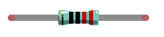# Control LED by Manual Button

## The example turns on an LED when you press the button. We connect two wires to the Arduino board for power up led and. this tutorial does not use for Arduino program to control ledFirst, try using the push button to make the LED blink manually.

### Component listArduino UNO x1 Buy NowBreadboard x1 Buy NowUSB cable x1 Buy NowLED x1 Buy NowResistor 220Ω x1 Buy NowPush button x1 Buy NowJumper M/M x2 Buy Now

## Circuit Knowledge

### Power supply

The power supply provides energy for the circuit, and it is divided into DC power and AC power. Voltage and current of DC power supply remain the same over time, such as the battery, power adapter. Voltage and current of  AC  power supply evolve periodically with time.  Its basic form is sinusoidal voltage(current). It is suitable for long-distance transmission of electric energy. And it is used to supply power to homes.Generally, electronic circuits use DC. Home appliances have rectifiers to convert AC into DC before they use them. Battery or battery pack can be represented by the following symbols:The positive and negative poles of the power supply can not be directly connected, otherwise, it may scald you and cause damage to the battery.

Voltage

The unit of voltage(U) is volt(V).

• 1kV=1000V
• 1V=1000mV
• 1mV=1000μV

Voltage is relative. As to a dry battery marked with "1.5V", it's positive (+) voltage is 1.5V higher than the negative (-) voltage. If you specify the negative as reference（0V），the positive voltage will be +1.5V.When two dry batteries are connected in series, the voltage of each point is as follows:In practical circuits, we usually specify negative as the reference voltage (0V), which is called "Ground". The positive is usually called "VCC". The positive and negative of the power supply is usually represented by two following symbols:Current (I)

The unit of current(I) is ampere(A).

• 1A=1000mA
• 1mA=1000μA.

A Closed-loop consisting of electronic components is necessary for current.

In the figure below: the left is a loop circuit, so current flows through the circuit. The right is not a loop circuit, so there is no current.#### Resistor

The unit of resistance(R) is the ohm(Ω).

• 1mΩ=1000kΩ
• 1kΩ=1000Ω.

The resistor is an electrical component that limits or regulates the flow of current in an electronic circuit. The left is the appearance of the resistor. and the right is the symbol of a resistor represented in the circuit.Color rings attached to the resistor is used to indicate its resistance. For more details of the resistor color code, please refer to the appendix of this book.

With the same voltage, there will be less current with more resistance. And the links among current, voltage and resistance can be expressed by the formula below: I=U/R.

In the following diagram,

the current through R1 is: I=U/R=5V/10kΩ=0.0005A=0.5mA.Do not connect the two poles of power supply with low resistance, which will make the current too high to damage electronic components.

## Component knowledge

Let us learn about the basic features of components to use them better.

### Jumper

The jumper is a kind of wire. It is designed to connect the components together with its two terminals by inserting it onto Breadboard or Arduino UNO.

Jumpers have a male end (pin) and female end (slot), so jumpers can be divided into the following 3 types.

 Jumper M/MJumper F/FJumper F/MThere are many small holes on the breadboard to connect Jumper.

Some small holes are connected inside the breadboard. The following figure shows the inner links among those holes.### Push button

Push-button has 4 pins. Two pins on the left are connected, and the right is similar to the left, which is shown in the below:When the push button is pressed, the circuit is turned on.

### LED

LED is a kind of diode. LED will shine only if the long pin of LED is connected to the positive electrode and the short pin is connected to the negative electrode.This is also the feature of the common diode. Diode works only if the voltage of its positive electrode is higher than its negative electrode.

The LED can not be directly connected to the power supply, which can damage components. A resistor with certain resistance must be connected in series in the circuit of LED.

### Circuit

In this experiment, the LED is controlled by the push button, and Arduino UNO here only plays the role of power supply in the circuit.

Firstly, connect components with jumpers according to "hardware connection". Secondly, check the connection to confirm there are no mistakes. Finally, connect the Arduino UNO board to the computer with a USB cable to avoid that touch on wires may cause short-circuit fault.Hardware connection

LED lights up when you press the push button, and it gets off when you release the button..0000000